# NEET and AIPMT NEET Physics Wave Optics MCQ Questions Solved

Watch Physics > Wave Optics Videos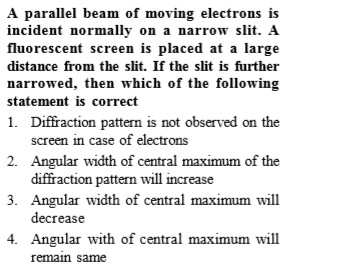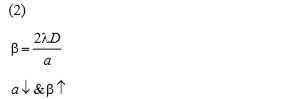Difficulty Level:

• 18%
• 52%
• 21%
• 11%
Crack NEET with Online Course - Free Trial (Offer Valid Till September 23, 2019)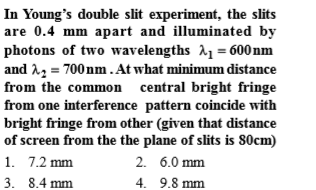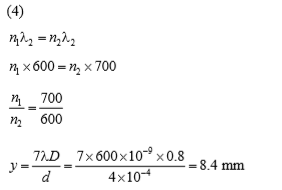Difficulty Level:

• 16%
• 36%
• 40%
• 10%
Crack NEET with Online Course - Free Trial (Offer Valid Till September 23, 2019)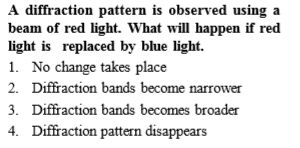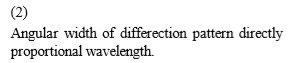Difficulty Level:

• 12%
• 61%
• 19%
• 10%
Crack NEET with Online Course - Free Trial (Offer Valid Till September 23, 2019)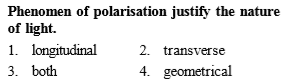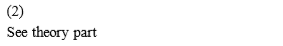Difficulty Level:

• 12%
• 57%
• 28%
• 5%
Crack NEET with Online Course - Free Trial (Offer Valid Till September 23, 2019)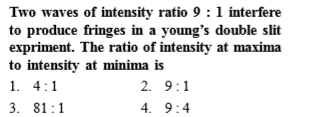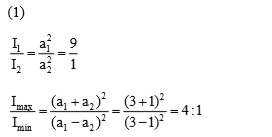Difficulty Level:

• 57%
• 16%
• 23%
• 6%
Crack NEET with Online Course - Free Trial (Offer Valid Till September 23, 2019)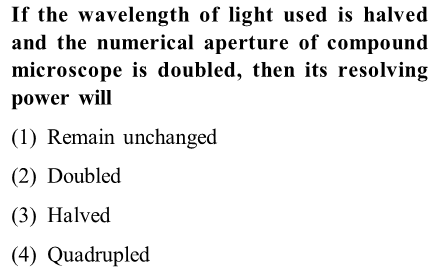(4)

Difficulty Level:

• 19%
• 32%
• 16%
• 35%
Crack NEET with Online Course - Free Trial (Offer Valid Till September 23, 2019)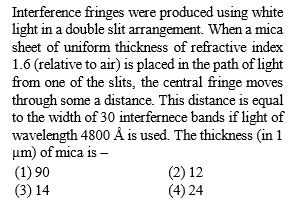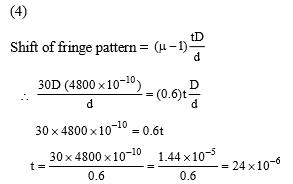Difficulty Level:

• 13%
• 29%
• 20%
• 40%
Crack NEET with Online Course - Free Trial (Offer Valid Till September 23, 2019)
NEET - 2017

Young's double slit experiment is first performed in air and then in a medium other than air. It is found that 8th bright fringe in the medium lies where 5th dark fringe lies in air. The refractive index of the medium is nearly

(a)1.25

(b) 1.59

(c) 1.69

(d) 1.78

(d) According to question, 5th fringe in air = 8th bright fringe in the medium

$\left(2×5-1\right)\frac{\lambda D}{2d}=8\frac{\lambda D}{\mu d}$

$9\frac{\lambda D}{2d}=8\frac{\lambda D}{\mu d}$

$\therefore$ Refractive index of the medium.

$\mu =\frac{16}{9}=1.7777=1.78$

Difficulty Level:

• 19%
• 35%
• 24%
• 25%
Crack NEET with Online Course - Free Trial (Offer Valid Till September 23, 2019)
NEET - 2017

Two polarids  are placed with their axis perpendicular to each other. Unpolarised light ${I}_{o}$ is incident on ${P}_{1}$. A third polaroid ${P}_{3}$ is kept in between  such that its axis makes an angle ${45}^{°}$ with that of ${P}_{1}$. The intensity of transmitted light through ${P}_{2}$

(a)$\frac{{I}_{o}}{2}$

(b) $\frac{{I}_{o}}{4}$

(c) $\frac{{I}_{o}}{8}$

(d) $\frac{{I}_{o}}{16}$

(c) According to the question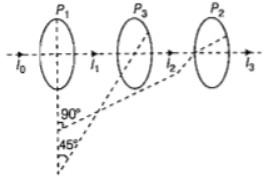From the above diagram, Intensity transmitted through${P}_{3}$

${I}_{2}=\frac{{I}_{o}}{2}{\mathrm{cos}}^{2}{45}^{°}$

Similarly, intensity transmitted through ${P}_{2}$

Difficulty Level:

• 15%
• 34%
• 43%
• 10%
Crack NEET with Online Course - Free Trial (Offer Valid Till September 23, 2019)
NEET - 2016

The interference pattern is obtained with two coherent light sources of intensity ratio n. In the interference pattern, the ratio $\frac{{I}_{max}-{I}_{min}}{{I}_{max}+{I}_{min}}$ will be

(a) $\frac{\sqrt{n}}{n+1}$             (b) $\frac{2\sqrt{n}}{n+1}$

(c) $\frac{\sqrt{n}}{{\left(n+1\right)}^{2}}$         (d) $\frac{2\sqrt{n}}{{\left(n+1\right)}^{2}}$

(b) It is given that $\frac{{l}_{2}}{{l}_{1}}=n⇒{l}_{2}=n{l}_{1}$

Ratio of intensites is given by

$\frac{{l}_{max}-{l}_{min}}{{l}_{max}+{l}_{min}}=\frac{{\left(\sqrt{{l}_{2}}+\sqrt{{l}_{1}}\right)}^{2}_{\left(\sqrt{{l}_{2}}-\sqrt{{l}_{1}}\right)}^{2}}{{\left(\sqrt{{l}_{1}}+\sqrt{{l}_{2}}\right)}^{2}+{\left(\sqrt{{l}_{2}}-\sqrt{{l}_{1}}\right)}^{2}}$

$=\frac{{\left(\sqrt{\frac{{l}_{2}}{{l}_{1}}}+1\right)}^{2}-{\left(\sqrt{\frac{{l}_{2}}{{l}_{1}}-1}\right)}^{2}}{{\left(\sqrt{\frac{{l}_{2}}{{l}_{1}}}+1\right)}^{2}+{\left(\sqrt{\frac{{l}_{2}}{{l}_{1}}-1}\right)}^{2}}$

$=\frac{{\left(\sqrt{n}+1\right)}^{2}-{\left(\sqrt{n}-1\right)}^{2}}{{\left(\sqrt{n}+1\right)}^{2}+{\left(\sqrt{n}-1\right)}^{2}}=\frac{2\sqrt{n}}{n+1}$

Difficulty Level:

• 10%
• 55%
• 21%
• 16%
Crack NEET with Online Course - Free Trial (Offer Valid Till September 23, 2019)